# Circular Motion

Uniform Circular Motion: - Circular motion is said to the uniform if the speed of the particle (along the circular path) remains constant.

Angular Displacement: -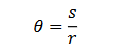Angular Velocity: -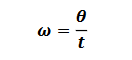Relation between linear velocity (v) and angular velocity (ω) is as 𝑣 = 𝑟𝜔

Angular Acceleration: -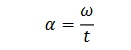Relation between linear velocity (v) and angular velocity (ω) as as 𝑎=𝑟𝛼

Centripetal Force: -

The force, acting along the radius towards the centre, which is essential to keep the body moving in a circle with uniform speed is called centripetal force. It acts always along the radius towards the centre. A centripetal force does no work.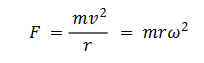Centrifugal Force: -

Centrifugal force is the fictitious force which acts on a body, rotating with uniform velocity in a circle, along the radius away from the centre. Magnitude of centrifugal force is,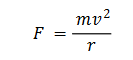Centripetal and centrifugal forces are equal in magnitude and opposite in direction. They cannot be termed as action and reaction since action and reaction never act on same body.

Banking of Roads: -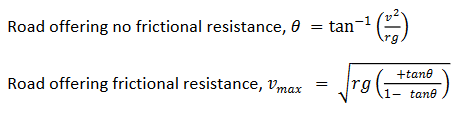Bending of Cyclist: -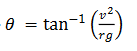(a) Velocity of the cyclist: -Greater the velocity, greater is his angle of inclination with the vertical.

(b) Radius of curvature: - Smaller the radius, greater is the angle with the vertical.

Time period of conical pendulum: -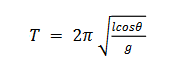Motion in a vertical circle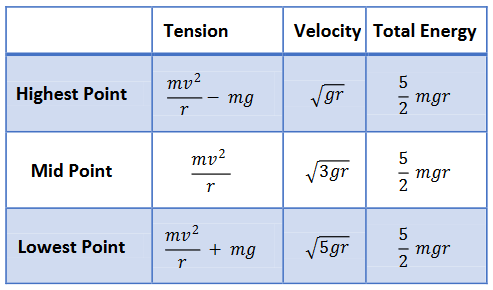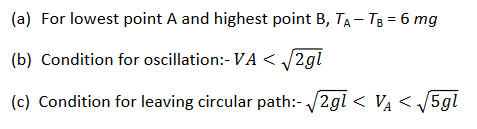Non-uniform circular motion: -

(a) The velocity changes both in magnitude as well as in direction.

(b) The velocity vector is always tangential to the path.

(c)The acceleration vector is not perpendicular to the velocity vector.

(d) The acceleration vector has two components.

(i) Tangential acceleration at changes the magnitude of velocity vector and is defined as,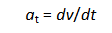(ii) Normal acceleration or centripetal acceleration ac changes the direction of the velocity vector and is defined as,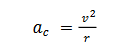(iii) The total acceleration is the vector sum of the tangential and centripetal acceleration.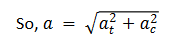Post By : Ravindra Yadav 03 Nov, 2019 2734 views Physics